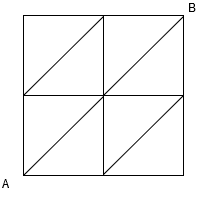GeeksforGeeks App
Open AppBrowser
Continue

# Adobe Interview Experience | MTS-1

## 1. Online Assessment Test (HackerRank)

a. 15 aptitude questions (15 mins)

b. 5 input-output programs (15 mins)

c. 2 programming questions (45 mins)

## 2. On-site Round 1 – Technical Round (1 Hour)

a. Discussion on projects, their architecture diagrams, etc.

b. Sort an array containing 0s and 1s in single pass, O(n) time and O(1) space complexity.

c. Add 1 to the number stored in a singly linked list form. Each digit is represented by a single node. Head of the linked list points to the most significant digit of the number. Do it in a single traversal.

d. How many different ways to form an N-bit binary number such that no two consecutive 1s occur together in N-bit form. For example, N = 3 then 101, 010, 000, 001, 100 are valid but 110, 111, 011 are invalid. Hence the output is 5. I wrote the program but interviewer told me that there is a math formula for this also.

e. Producer – Consumer problem (pseudo-code for this problem), semaphore and mutex, critical section, etc. Some questions on wait() and signal() like when will be the deadlock in different cases etc.

## 3. On-site Round 2 – Technical Round (1 Hour)

Discussion on a project which the interviewer smartly converted into the problem and asked many follow up questions on the same problem.

Problem – How will you represent an image in memory? (i.e. by a matrix with each cell as a pixel).

So consider there is a monochrome image represented as your matrix, how will you draw a line-segment on your same matrix programmatically? (which means filling cells with a number from which that line segment will pass).

Remember that a line segment can be an aligned line to X-axis or Y-axis and can be a non-aligned line. Write a program for that.

I assumed that end-points of the line-segment are integers in 2D space and wrote the code accordingly but interviewer then asked me whether this code will work if the end-points are float/double and had a discussion on that to make it a generalized solution.

Following this, the interviewer asked some boundary cases like what if the end-points of line-segment are not inside the matrix or line-segment is partially inside the matrix.

Considering that line-segment is not aligned to any axis, how will you find the point where the line-segment first enters/exits the matrix if the line-segment is not bounded by the matrix?

## 4. On-site Round 3 – Technical Round (1 Hour)

a. Some problems on pointers like is the difference between pointers valid or not, problems on pass by reference to a function, etc.

b. What happens when we declare an object of a class and allocate memory to it?

c. Questions on inheritance, polymorphism, virtual function, etc.

d. Write a program to implement a Hash Table with the following APIs-

i. void insert(int key, int value),
ii. void delete(int key),
iii. int search(int key) and
iv. int getRecentElement() (Recent element is that element which has been touched by either insert() or search(), return its key).

All the above APIs should work in O(1). Assuming no duplicate keys.

e. Search an element in an array where each consecutive pair of element differ exactly by 1 i.e |A[i] – A[i+1]| = 1 and |A[i-1] – A[i]| = 1.

f. Given N threads and single instances of N different types of resources, how will you allocate the resources to threads such that there is no deadlock and waiting time is minimum? Assume that each thread can come at any time and ask for any number of resources. You don’t know when a thread will come.

## 5. On-site Round 4 – Director Round (1 Hour)

a. Project discussion.

b. How will you identify whether software that you are going to install in your PC is a malware? Give some ways to identify before installation and after installation of that software.

c. How will you identify whether this software has deleted/added some files to your file system? I said we can compare the tree structure of the file system before and after installing the software.

d. Given two files containing a list of file names present before and after the installation. How will you find out the difference between the two files? Write pseudocode for fileDifference(File A, File B).

e. You can move Up (?), Right(?) and Up-Right(?), how many ways to reach the top-right corner from the bottom-left corner of a N x N matrix? Matrix representation is –I gave my approach using dynamic programming but the interviewer told me to derive a mathematical formula (which is Delannoy Number, http://mathworld.wolfram.com/DelannoyNumber.html).

## 6. On-site Round 5 – Manager Round (1 Hour)

a. Program to convert a number into English Word Format (Indian Currency). For example – 1, 23, 500 to “One Lakh Twenty Three Thousand Five Hundred”, 100 to “One Hundred” etc.

https://www.geeksforgeeks.org/convert-number-to-words/

b. Program to check if two trees are a mirror image of each other or not.

## Interview Preparation Resources

### 3. OOP Concepts

#### a. https://www.geeksforgeeks.org/oops-object-oriented-design/b. https://www.geeksforgeeks.org/c-plus-plus/

My Personal Notes arrow_drop_up